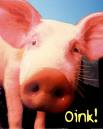Pet Pigs     |     Dogs     |     Cats     |     Horses     |    Birds   |     Small Pets
 How you can tell the Weight of a Pet PigHelp Rescue Homeless Pets with a Gift of One Dollar

It is often difficult to get a pig to stand still long
enough to get its accurate weight. By using the simple
formula below you can get a pretty good estimate. It will be within
about three percent of the actual weight, of how much your
pig weighs without using a scale.

You will need some cloth tape commonly used by tailors.

Below is the formula:

1. First, take the measurement around the girth (or just
behind the front legs.) You need a cloth tape long enough to
go around your pig's girth and over the shoulders.

2. Multiply the Girth measurement by itself (square the
measurement).

3. Next, Measure the length of your pig from the top of the
head between the ears to the base of the tail.

4. Take the measurement of the girth that has been
multiplied by itself and multiply by the pig's length.

5. Take the result of the squared girth measurement and the
length measurement and divide the result by 400.

You will have an accurate weight estimate within three
percent of the actual weight.

For example, if your pig's girth is 45 inches around: 45 X
45 = 2025. Your pig's length is 43 inches: 2025 X 43 =
87,075. 87,075/400 = 217.6875

The variance of three percent for the weight of 217 is 6.51,
meaning that my example pig weighs between 211.1775 and
224.1975. Essentially between 211 and 224 pounds.

This won't help you if you need an exact weight for show,
especially if your pig is on the cusp of a particular weight
class. But for general reasons like for your veterinarian or
just to sell your pig this is accurate enough for most
cases.

Credit for this formula goes to the Old Farmer's Almanac 1993

Fun Filled Plush Pigs

Custom Search